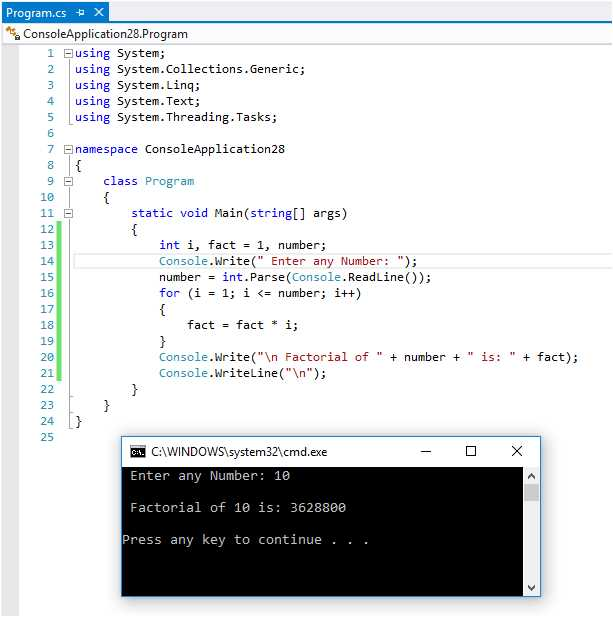#### What is a Factorial program in C#

Total Post:46

Points:324

651  View(s)
Ratings:
Rate this:

Like -

4! = 4*3*2*1 = 24

6! = 6*5*4*3*2*1 = 720

1.##### Re: What is a Factorial program in C#

Factorial program in c#...

The factorial is normally used in Combinations and Permutations (mathematics).

```using System;
using System.Collections.Generic;
using System.Linq;
using System.Text;

namespace ConsoleApplication28
{
class Program
{
static void Main(string[] args)
{
int i, fact = 1, number;
Console.Write(" Enter any Number: ");
for (i = 1; i <= number; i++)
{
fact = fact * i;
}
Console.Write("\n Factorial of " + number + " is: " + fact);
Console.WriteLine("\n");
}
}
}
```Thank You...!!